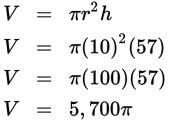# SAT Math Multiple Choice Question 564: Answer and Explanation

### Test Information

Question: 564

9. A grain producer is filling a cylindrical silo 20 feet wide and 60 feet tall with wheat. Based on past experience, the producer has established a protocol for leaving the top 5% of the silo empty to allow for air circulation. Assuming the producer follows standard protocol, what is the maximum amount of wheat that should be put in the silo?

• A. 5,144π ft3
• B. 5,700π ft3
• C. 20,577π ft3
• D. 22,800π ft3

Explanation:

B

Difficulty: Medium

Category: Additional Topics in Math / Geometry

Strategic Advice: Check the formula page at the beginning of the Math section—the formula for finding the volume of a cylinder is V = πr 2h.

Getting to the Answer: Leaving the top 5% of the silo empty is another way of saying that the silo should only be filled to 95% of its total height, so multiply the height (60 ft) by 0.95 to get 57 ft and then find the volume. Don't forget to divide the width of the silo (20 ft) by 2 to find the radius: